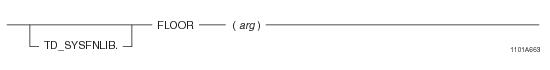# 15.00 - FLOOR - Teradata Database

## Teradata Database SQL Functions, Operators, Expressions, and Predicates

Product
Release Number
15.00
Content Type
Programming Reference
Publication ID
B035-1145-015K
Language
English (United States)

## FLOOR

### Purpose

Returns the largest integer equal to or less than the input argument.

### Syntaxwhere:

 Syntax element… Specifies… TD_SYSFNLIB the name of the database where the function is located. arg a numeric expression.

### ANSI Compliance

This is a Teradata extension to the ANSI SQL:2011 standard.

### Invocation

FLOOR is an embedded services system function. For information on activating and invoking embedded services functions, see “Embedded Services System Functions” on page 24.

### Argument Types and Rules

Expressions passed to this function must have one of the following data types:

BYTEINT, SMALLINT, INTEGER, BIGINT, FLOAT/REAL/DOUBLE PRECISION, DECIMAL/NUMERIC, or NUMBER

### Return Value

FLOOR is a scalar function that returns the following values:

 IF arg is... THEN FLOOR returns... a non-exact number the next largest integer that is equal to or less than arg. an exact number the input argument arg. NULL NULL.

### Result Type and Attributes

The result type is the same data type as that of the numeric input argument.

If the input argument is defined as a DECIMAL/NUMERIC with a precision less than 38, the return DECIMAL/NUMERIC value will have its precision increased by 1. For example, if DECIMAL(6,4) were passed in, it would be increased and returned as a DECIMAL(7,4). If the precision is 38, the scale will be reduced by 1 unless the scale is 0. For example, a DECIMAL(38,38) results in a return data type of DECIMAL(38,37).

The default title for FLOOR is FLOOR(arg).

For information on default data type formats, see SQL Data Types and Literals.

### Example

The following query returns the FLOAT value 13E0, since 13 is the largest integer that is less than the FLOAT value 13.6E0.

`   SELECT FLOOR (136E-1);`

### Example

The following query returns a DECIMAL value of -7.0 since -7 is the largest integer that is less than the DECIMAL literal -6.5.

`   SELECT FLOOR(-6.5);`

### Example

The following query returns a value of -7.0 with a data type of DECIMAL(3,1), since -7 is the largest integer less than DECIMAL -6.5. Note that the precision of the return value is increased by 1.

`   SELECT FLOOR (CAST(-6.5 AS DECIMAL(2,1)));`